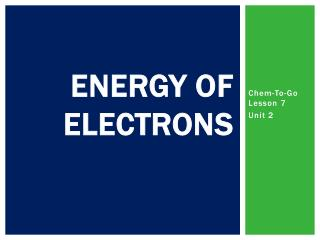DownloadDownload PresentationEnergy of Electrons

# Energy of Electrons

Télécharger la présentation## Energy of Electrons

- - - - - - - - - - - - - - - - - - - - - - - - - - - E N D - - - - - - - - - - - - - - - - - - - - - - - - - - -
##### Presentation Transcript

1. Energy of Electrons Chem-To-Go Lesson 7 Unit 2

2. Energy basics • Four Important Wave Words: • Wavelength (λ) • Frequency (ν) • Energy (E) • Speed of light (c) Two Important Equations: c = λν E = hν c = 3.0x108 m/s h= 6.626x10-34 J·s • All energy travels in the form of a wave. • Scientists measure the wavelength of a wave to identify its type of energy.

3. Energy basics c = 3.0x108 m/s for ALL • The spectrum includes all forms of energy. We’ll focus mainly on the VISIBLE SPECTRUM. • Notice that RED has the longest λ but the lowest v. • Notice that VIOLET has the shortest λ but the highest v.

4. Connection to atomic structure • ABSORPTION • Atoms, specifically the electrons, can absorb photons of energy. • With higher energy, the electron must move from its normal energy level [ground state] to a higher energy level in the electron cloud [excited state]. • The excited state is temporary. PHOTON – a quantum or “piece” of energy GROUND STATE – lowest allowable energy level for a certain electron EXCITED STATE – temporary high energy level for a certain electron

5. Connection to atomic structure • EMISSION • Eventually, the electron must release [emit] the energy that it has recently absorbed. • The emitted photon of energy will leave the atom in the form of a light wave. • If the wave’s wavelength falls between 400 and 700 nm, then it will be visible light. This emission of light in the visible spectrum is how we create fireworks.

6. Useful to calculate the energy • Useful to know how much energy is coming outof an atom • Useful to know how much energy to put intoan atom to achieve a particular color c = λν E = hν h= 6.626x10-34 J·s c = 3.0x108m/s

7. Calculating the energy, wavelength, or frequency of light • An atom emits a photon of green light with wavelength of 650 nm. How much energy does the photon carry? c c= 3.0x108 m/s E h= 6.626x10-34 J·s